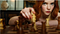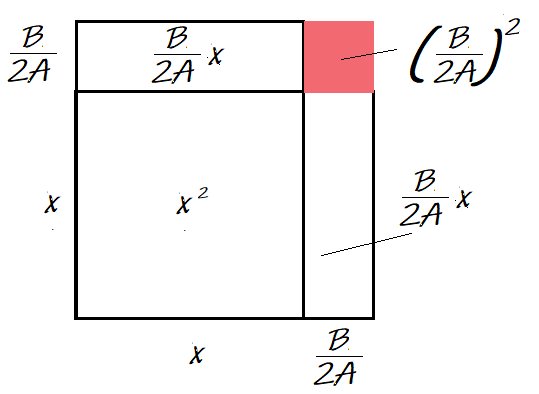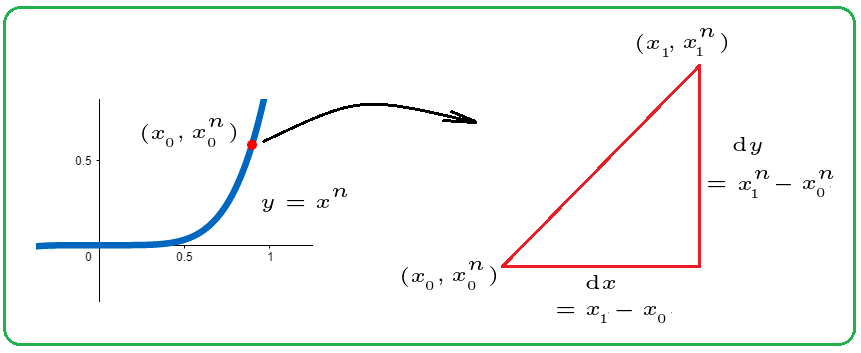# Oops.

## How to fail at maths.

Have you seen my latest blunder? Here it is:

Can You Solve This Limit?

To magnify my shame, here is the video version:

The goal was to solve this limit:Anya Taylor-Joy as Chess Grandmaster Beth Harmon (Netflix, “The Queen’s Gambit)

# I Checkmated Beth Harmon

## And you can too.

If you have yet to see the new Netflix mini-series, The Queen’s Gambit, worry not. This article contains (almost) no spoilers.

# Can You SolveThis Limit?

## Try it out. Just for fun.

YouTube math aficionado, Dr. Peyam has published a video featuring this limit:

# What is the Point of Maths?

## Are you satisfied with the answer in the back of the book?

I received an email the other day — a response to the antiderivative challenge. Enjoy it. And ask yourself: what does maths do for you?

What is it that drives folks … to delight in taking five lines to solve a maths question that we could solve in one line?

# Antiderivative — The wrong way

Is it possible to solve ∫(1/t)dt using the reverse power law?

We can write the problem as follows:

# The Power Rule from First Principles

## A little geometry; a few infinitesimals

The Power Rule for derivatives is one of the first tricks we learn in Calculus I. It’s such a refreshing alternative to using the limit definition.

Let’s start with a question. For the graph of xⁿ, what is the slope at a given point?

For example, f(x) =x². We can represent f(x) as the area of a square with sides, x. We increase each side by an infinitesimal, dx.

# Can You Solve the ∫ x² dx Challenge?

## Solve this simple integral without using the reverse power rule.

Yesterday (October 30, 2020), YouTuber Steven Chow (blackpenredpen) posted a video with this goal: Integrate x² without the power rule. Before seeing Steve’s solution, I posted my own video. (I didn’t want to be unduly influenced.)

Have a crack at it yourself. Then see how I solved it (below).# Completing the Square — a Visual Intuition

## Wherein we discover the roots of the quadratic formula

You want to find the roots of a quadratic polynomial. Good news! The method of completing the square always works. What makes that so, and what other information can we glean from this method?

Let’s find out with an example. We’ll find the roots of:

# The Squeeze Theorem Visualized

## A Geometric Illustration

The best way to define the Squeeze Theorem is with an example. We’ll use it to prove the following identity:

# The Product Rule — an Intuition

## More visual calculus for you

How do we take the derivative of a product of functions? You may have memorized this formula:# The Power Rule: An Infinitesimal Approach

## This is the derivation you probably didn’t get in Calculus I

This derivation of the power rule does not use limits. Its inspiration is Abraham Robinson’s non-standard analysis and hyper-real numbers.

If you learned a derivation of the power rule, it probably started something with a definition of the derivative: